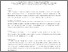# Efficient hybrid algorithms to solve mixed discrete-continuous optimization problems: A comparative study

Liu, Dianzi, Liu, Chengyang, Zhang, Chuanwei, Xu, Chao, Du, Ziliang and Wan, Zhiqiang (2018) Efficient hybrid algorithms to solve mixed discrete-continuous optimization problems: A comparative study. Engineering Computations, 35 (2). pp. 979-1002. ISSN 1758-7077Preview PDF (Accepted manuscript) - Accepted Version Download (556kB) | Preview

## Abstract

Purpose: – In real world cases, it is common to encounter mixed discrete-continuous problems where some or all of the variables may take only discrete values. To solve these non-linear optimization problems, it is very time-consuming in use of finite element methods. The purpose of this paper is to study the efficiency of the proposed hybrid algorithms for the mixed discrete-continuous optimization, and compares it with the performance of Genetic Algorithms (GA). Design/methodology/approach: – In this paper, the enhanced multipoint approximation method (MAM) is utilized to reduce the original nonlinear optimization problem to a sequence of approximations. Then, the Sequential Quadratic Programming (SQP) technique is applied to find the continuous solution. Following that, the implementation of discrete capability into the MAM is developed to solve the mixed discrete-continuous optimization problems. Findings: – The efficiency and rate of convergence of the developed hybrid algorithms outperforming GA are examined by six detailed case studies in the ten-bar planar truss problem and the superiority of the Hooke-Jeeves assisted MAM algorithm over the other two hybrid algorithms and GAs is concluded. Originality/value: – The authors propose three efficient hybrid algorithms: the rounding-off, the coordinate search, and the Hooke-Jeeves search assisted MAMs, to solve nonlinear mixed discrete-continuous optimization problems. Implementations include the development of new procedures for sampling discrete points, the modification of the trust region adaptation strategy, and strategies for solving mix optimization problems. To improve the efficiency and effectiveness of metamodel construction, regressors φ defined in this paper can have the form in common with the empirical formulation of the problems in many engineering subjects.

Item Type: Article discrete-continuous design optimization,hybrid algorithms,multipoint approximation method,direct search Faculty of Science > School of Mathematics Pure Connector 08 Jan 2018 10:00 22 Oct 2022 03:27 https://ueaeprints.uea.ac.uk/id/eprint/65873 10.1108/EC-03-2017-0103View Item